Ex 5.2

Chapter 5 Class 6 Understanding Elementary Shapes
Serial order wise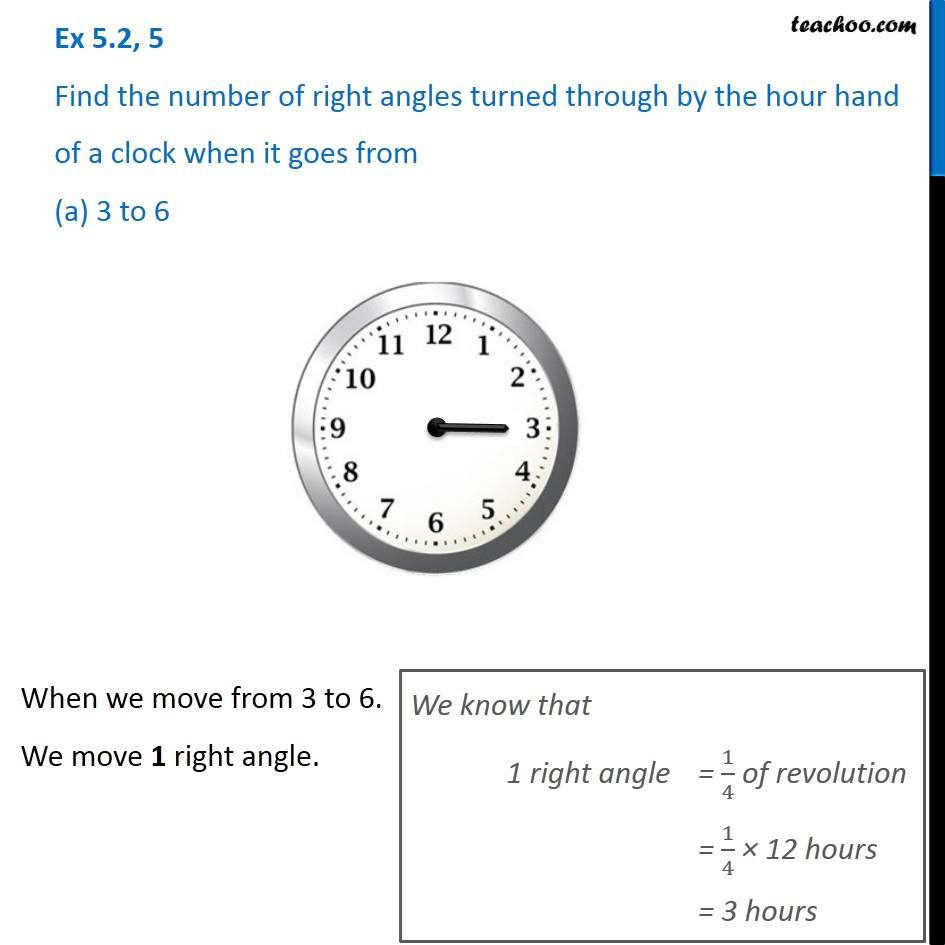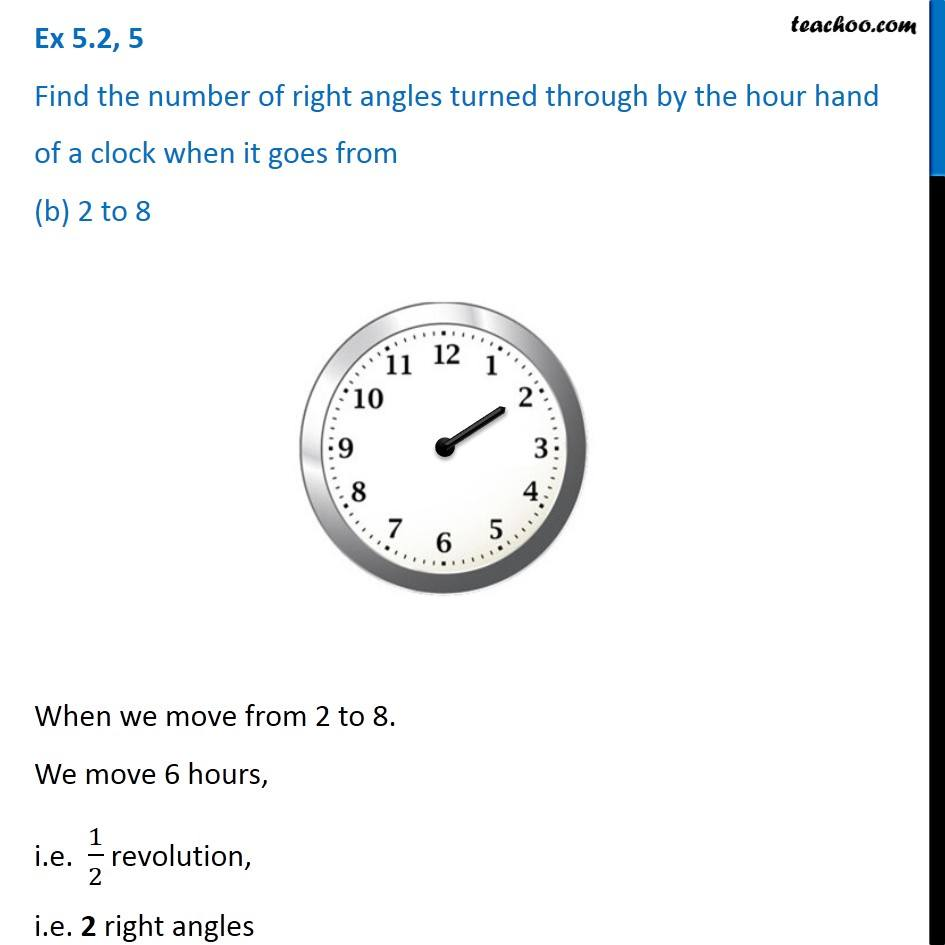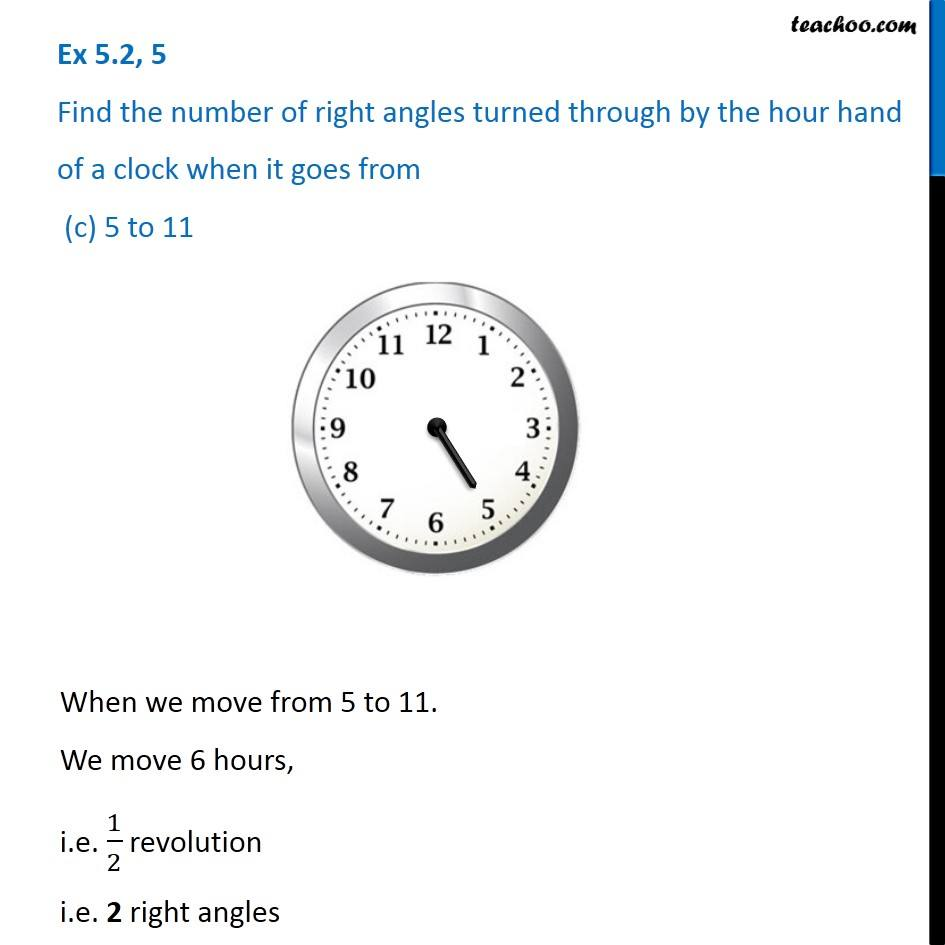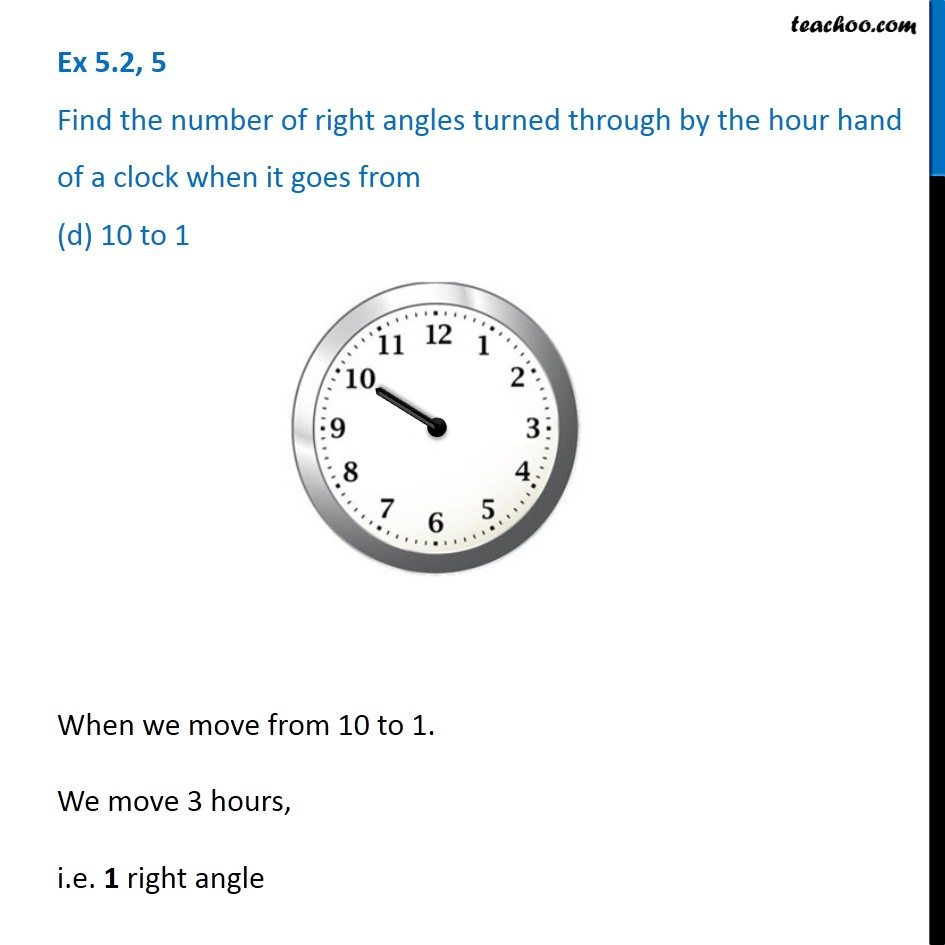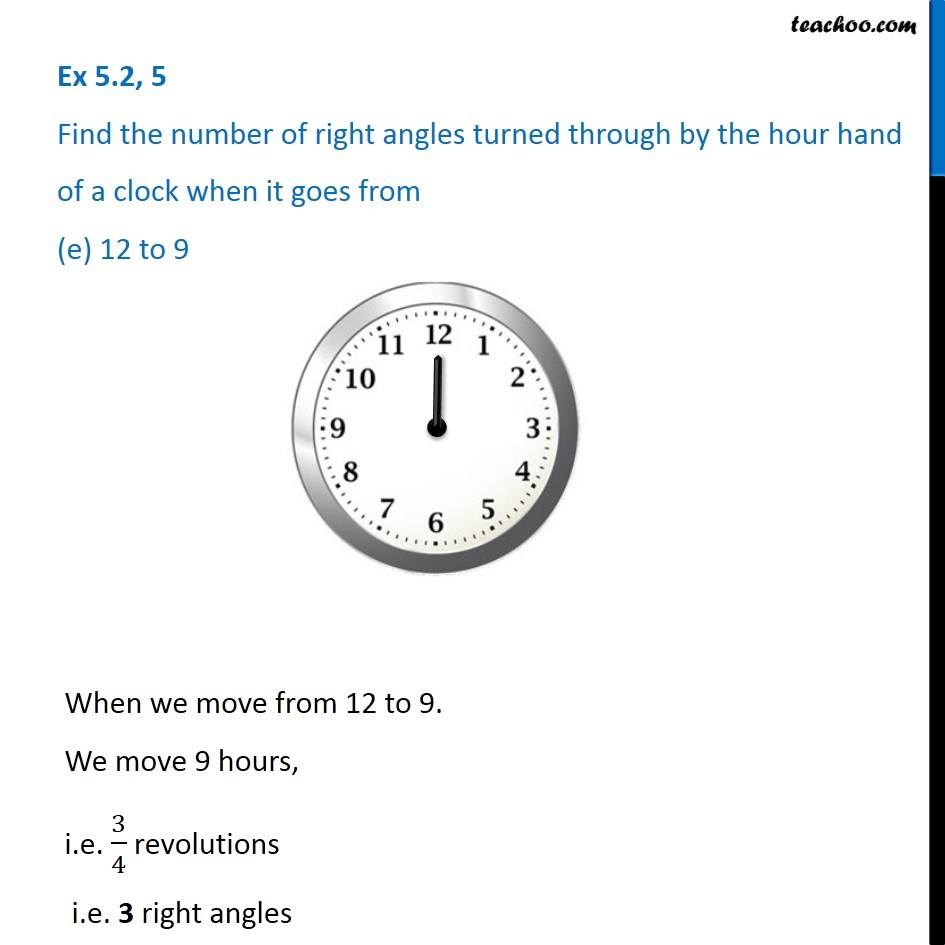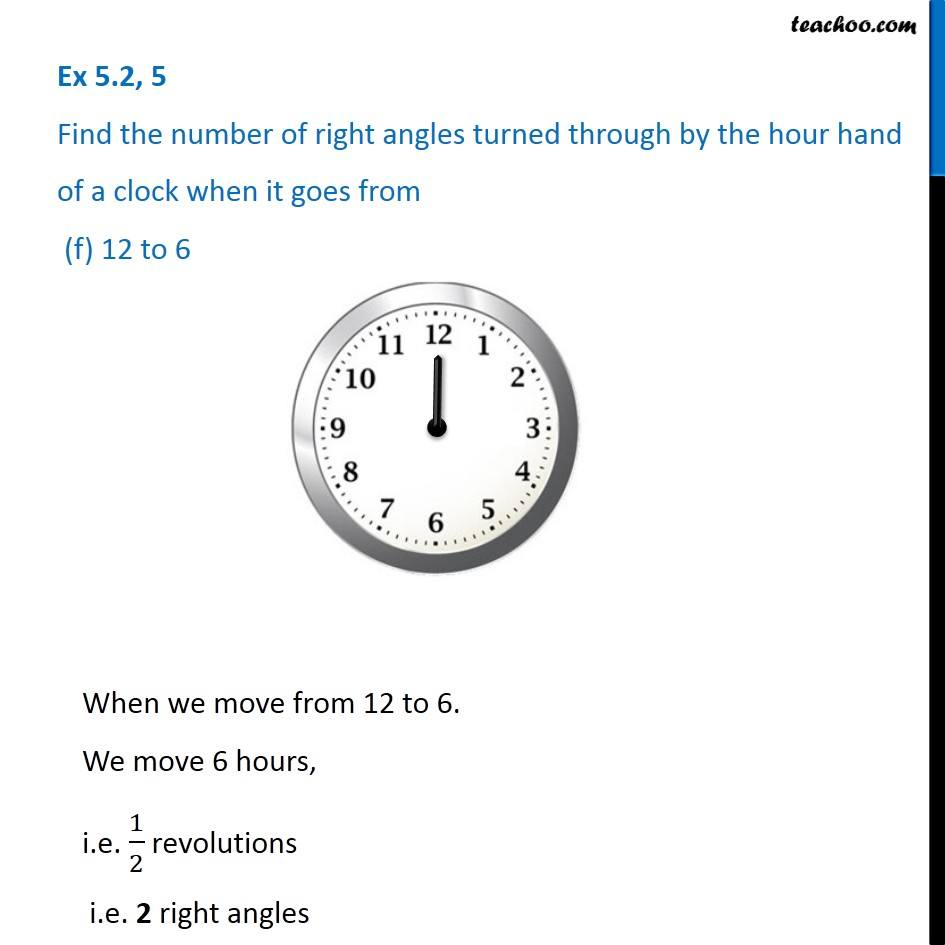Learn in your speed, with individual attention - Teachoo Maths 1-on-1 Class

### Transcript

Ex 5.2, 5 Find the number of right angles turned through by the hour hand of a clock when it goes from (a) 3 to 6 When we move from 3 to 6. We move 1 right angle. We know that 1 right angle = 1/4 of revolution = 1/4 × 12 hours = 3 hours Ex 5.2, 5 Find the number of right angles turned through by the hour hand of a clock when it goes from (b) 2 to 8 When we move from 2 to 8. We move 6 hours, i.e. 1/2 revolution, i.e. 2 right angles Ex 5.2, 5 Find the number of right angles turned through by the hour hand of a clock when it goes from (c) 5 to 11 When we move from 5 to 11. We move 6 hours, i.e. 1/2 revolution i.e. 2 right angles Ex 5.2, 5 Find the number of right angles turned through by the hour hand of a clock when it goes from (d) 10 to 1 When we move from 10 to 1. We move 3 hours, i.e. 1 right angle Ex 5.2, 5 Find the number of right angles turned through by the hour hand of a clock when it goes from (e) 12 to 9 When we move from 12 to 9. We move 9 hours, i.e. 3/4 revolutions i.e. 3 right angles Ex 5.2, 5 Find the number of right angles turned through by the hour hand of a clock when it goes from (f) 12 to 6 When we move from 12 to 6. We move 6 hours, i.e. 1/2 revolutions i.e. 2 right angles